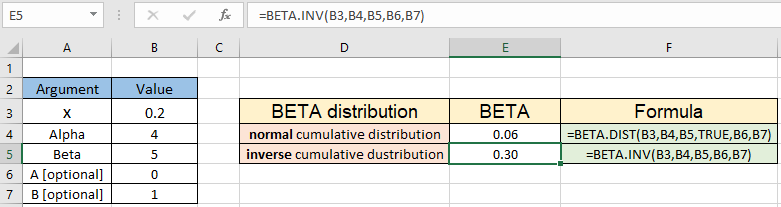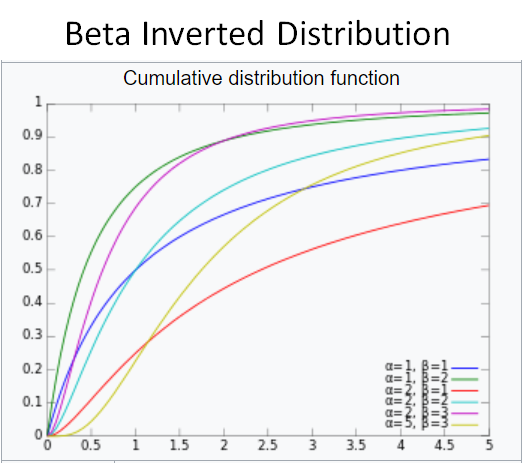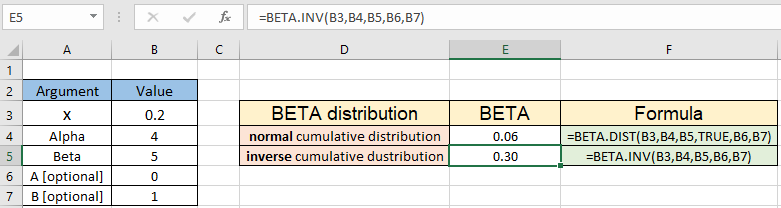# How to use the BETA.INV function in ExcelIn this article, we will learn How to use the  function in Excel.

What is Beta distribution and inverse beta distribution?

Beta Distribution is a continuous probability distribution function having a couple of parameters also known as alpha and beta. Generally, beta distribution is used to model one's uncertainty about the probability of success of an experiment. Whereas Inverted Beta cumulative distribution is also known as beta prime distribution. The beta prime distribution is the distribution of the odds ratio associated with a random variable with the beta distribution. Since variables with beta distributions are often used to model random probabilities and proportions, the corresponding odds ratios occur naturally as well. How beta distribution behaves with changing alpha and beta values for the inverted beta or Beta prime cumulative distribution shown below.BETA.INV Function in Excel

The Excel BETA.INV function calculates the inverse of the cumulative beta distribution function or the probability density function of the Beta distribution, for a supplied set of parameters. Let's learn these parameters below.

BETA.INV Function syntax:

 =BETA.INV(x, alpha, beta, [A], [B])

x : value between A and B at which to evaluate the function

alpha : first parameter of the distribution

beta : second parameter of the distribution

[A] : [Optional] lower bound to the interval of x. Default is 0

[B] : [Optional] upper bound to the interval of x. Default is 1

Example :

All of these might be confusing to understand. Let's understand how to use the function using an example. Here we have the parameters for a distribution and we need to find the beta cumulative distribution function

Use the formula:

 =BETA.INV(B3,B4,B5,B6,B7)As you can see, the inverse of beta distribution for the variable x ( = 0.2 ) comes out to be 0.06 whereas the normal beta distribution function returns the 0.30 for the same parameters.

Here are all the observational notes using the BETA.INV function in Excel
Notes :

1. The function only works with numbers. If any argument is nonnumeric, BETA.INV returns the #VALUE! error value.
2. Arguments to function can be fed directly or using the cell reference.
3. If optional argument [A] and [B] are omitted. Default values are taken as 0 and 1. So x must lie between 0 and 1.
4. The function returns #NUM error value :
1. If alpha <= 0 or beta <= 0
2. If x < A, x > B, or A = B

Hope this article about How to use the BETA.INV function in Excel is explanatory. Find more articles on statistical formulas and related Excel functions here. If you liked our blogs, share it with your friends on Facebook. And also you can follow us on Twitter and Facebook. We would love to hear from you, do let us know how we can improve, complement or innovate our work and make it better for you. Write to us at info@exceltip.com.

Related Articles :

How to use the VAR function in Excel : Calculate the variance for the sample dataset in excel using the VAR function in Excel.

How to Calculate Standard Deviation in Excel : To calculate the standard deviation we have different functions in Excel. The standard deviation is the square root of the variance value but It tells more about the dataset than variance.

Regressions Analysis in Excel : Regression is an Analysis Tool, which we use for analyzing large amounts of data and making forecasts and predictions in Microsoft Excel.

How to Create Standard Deviation Graph : The standard deviation tells how much data is clustered around the average of the data. Learn how to create a standard deviation graph here.

How to use Excel NORMDIST Function : Calculate the Z score for the normal cumulative distribution for the pre specified values using the NORMDIST function in Excel.

How to use Excel NORM.INV Function : Calculate the inverse of Z score for the normal cumulative distribution for the pre-specified probability values using the NORM.INV function in Excel.

Popular Articles :

How to use the IF Function in Excel : The IF statement in Excel checks the condition and returns a specific value if the condition is TRUE or returns another specific value if FALSE.

How to use the VLOOKUP Function in Excel : This is one of the most used and popular functions of excel that is used to lookup value from different ranges and sheets.

How to use the SUMIF Function in Excel : This is another dashboard essential function. This helps you sum up values on specific conditions.

How to use the COUNTIF Function in Excel : Count values with conditions using this amazing function. You don't need to filter your data to count specific values. Countif function is essential to prepare your dashboard.

Terms and Conditions of use

The applications/code on this site are distributed as is and without warranties or liability. In no event shall the owner of the copyrights, or the authors of the applications/code be liable for any loss of profit, any problems or any damage resulting from the use or evaluation of the applications/code.